« Example 4.2.2-1 Example 4.2.2-3 »

Example 4.2.2-2

(project <Β_422-2>)

The structure of this example derives from 1st example by removing the two middle beams.

The aspect ratio for both slabs is ε=Ly/Lx=12.0/4.0=3.0> 2.0, therefore they can be treated and analysed as two continuous one-way slabs. However, each one of them is supported on four edges and therefore at the up and down supports they behave as two-way slabs. This behaviour will be examined next.

For 1.00 m wide slab strip:

Self-weight: go=0.15m·1.00m·25.00 kN/m3= 3.75 kN/m, Covering load: ge=1.00 kN/m (given),

Thus, the total load is p=γg·g+γq ·q=1.35x4.75+1.50x5.00=13.9 kN/m. The bending moment at the support is calculated using table b3, line 1.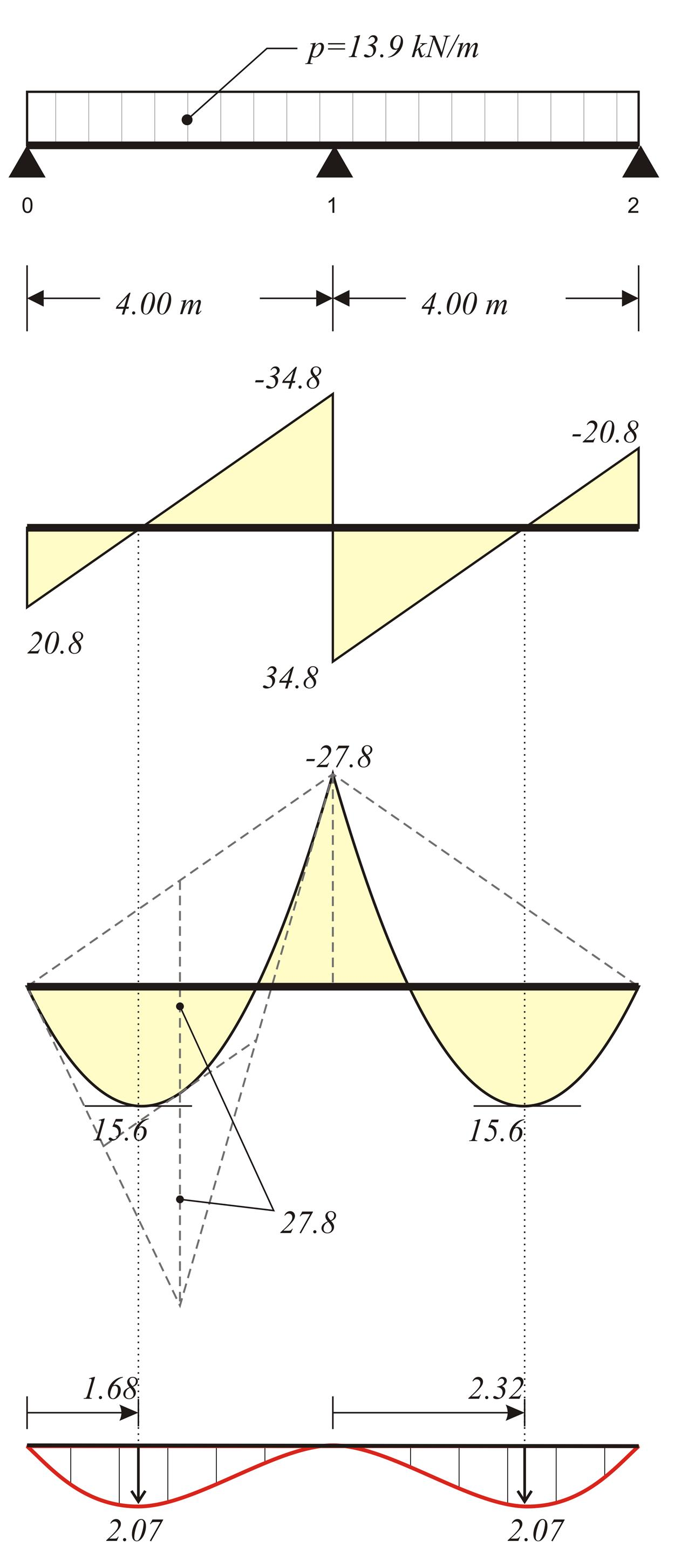Figure 4.2.2-23 Figure 4.2.2-23 M1=-p · L2/8=-13.9x4.02/8=-27.8 kNm , V01=p · L/2+M1/L=13.9x4.0/2-27.8/4.0= =20.8 kN V10= p · L/2-M1/L=13.9x4.0/2+27.8/4.0= =34.8 kN maxM01=V012/(2 · p)=20.82/(2x13.9)= =15.6 kNm According to §4.5.2, from expression (3) → C1=(-13.9x4.03/24+20.8x4.02/6)m2= =18.4 kN · m2 Expression (4) → (13.9/6)z3-(20.8/2)z2-0+18.4=0 → 2.317z3-10.4z2+18.4=0 → zmax=1.68 m (2) → y(z)=1/9.225x[(13.9/24)x1.684-(20.8/6)x1.683+0x1.682+18.4x1,68)] → y(1.68)=2.07 mm

Before analysing, set these parameters, as in 1st example: Tab Meshing: "Overall size" = 0.10 m, "Perimeter size" = 0.05 m, Tab Modules: "SLABS" = ON, Tab Loads: "Adverse Slabs" = ΟΝ.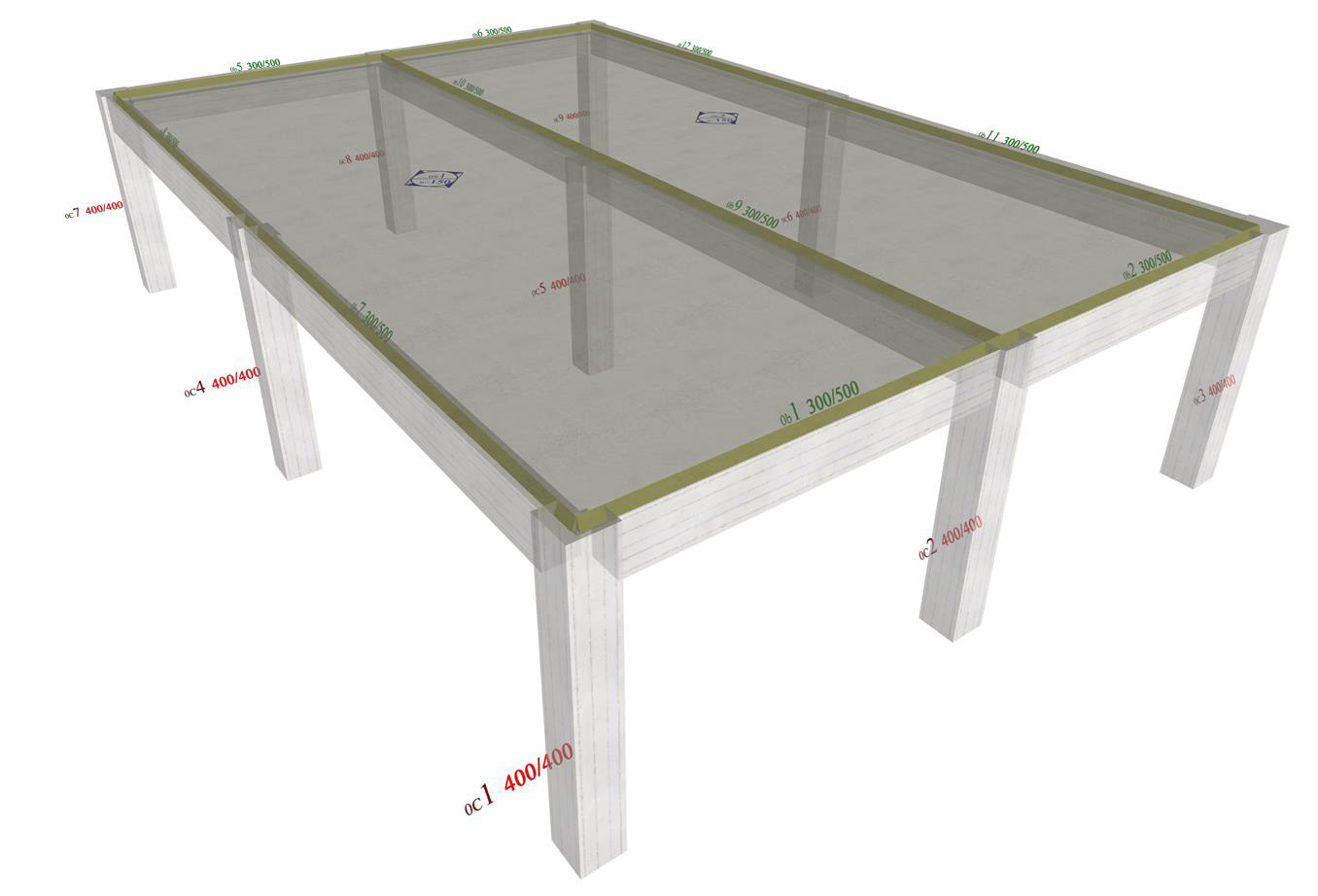Figure 4.2.2-24: After the analysis, the real structure in 3D is displayed.
Figure 4.2.2-24: After the analysis, the real structure in 3D is displayed.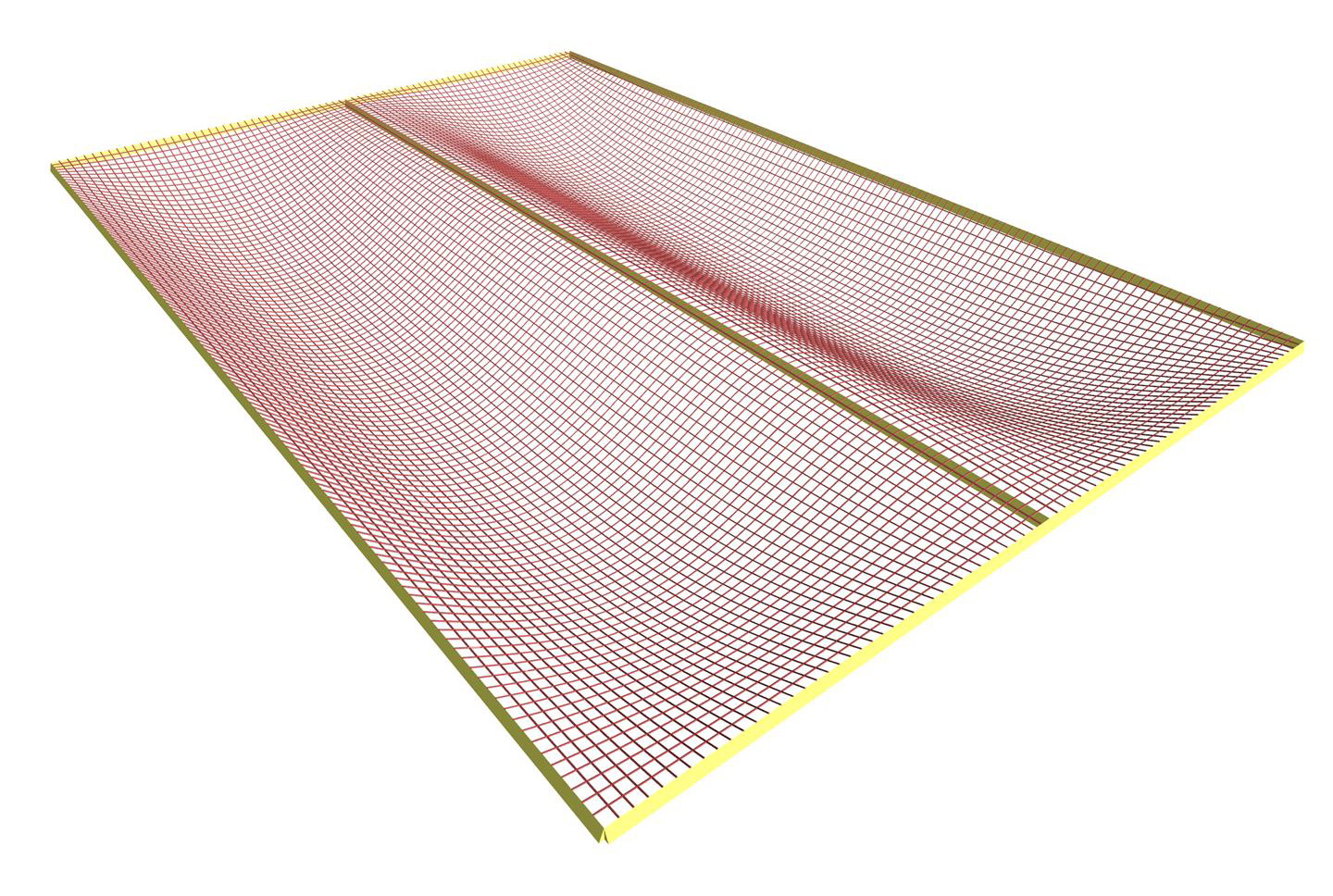Figure 4.2.2-25: The deformation of slabs in 3D.
Figure 4.2.2-25: The deformation of slabs in 3D.

For most of the region towards direction y, the slab is curved only along direction x.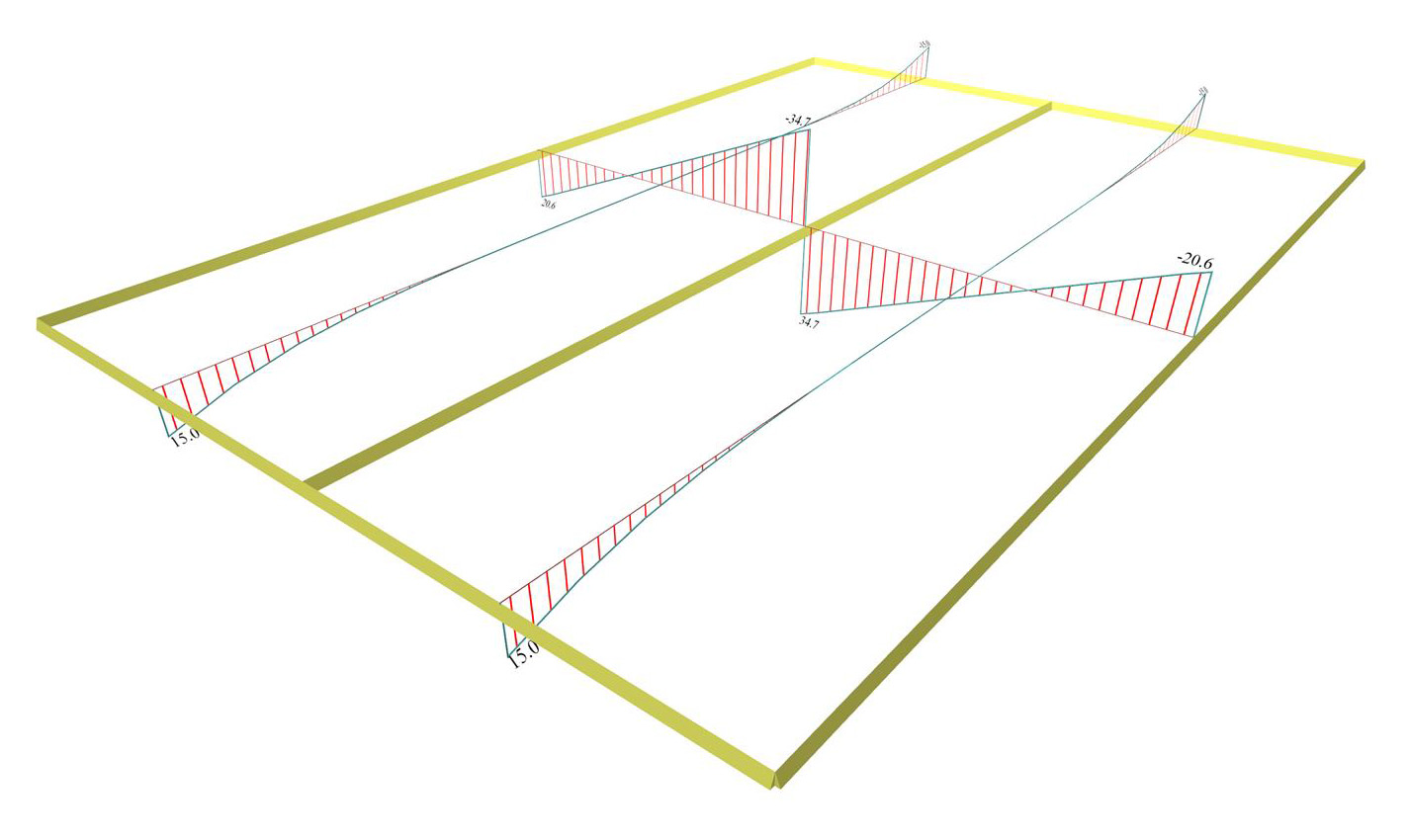Figure 4.2.2-26: Shear forces from Slab Results
Figure 4.2.2-26: Shear forces from Slab Results

One notices that in the middle cross-section, the values of shears [Vx] 20.6 and 34.7 kN, are equal to the respective values of the one-way slabs, calculated previously.

Also, shears [Vy] extend in an area near the supports with maximum value of 15.0 kN.

In general, at every single position of the diagrams, the value of shear forces and bending moments, obtained from the Slab Results section, is calculated by integrating the stress resultants of finite elements on a section with total width of 1.0 m across the position. The respective value, obtained from the FEM Results section, represents directly the stress resultants of the finite elements. More details are given in the §4.1.1.4.

In this case, the value of shear [Vy], in the middle cross-section, is 15.0 kN and 15.3 kN for Slab Results and FEM Results respectively.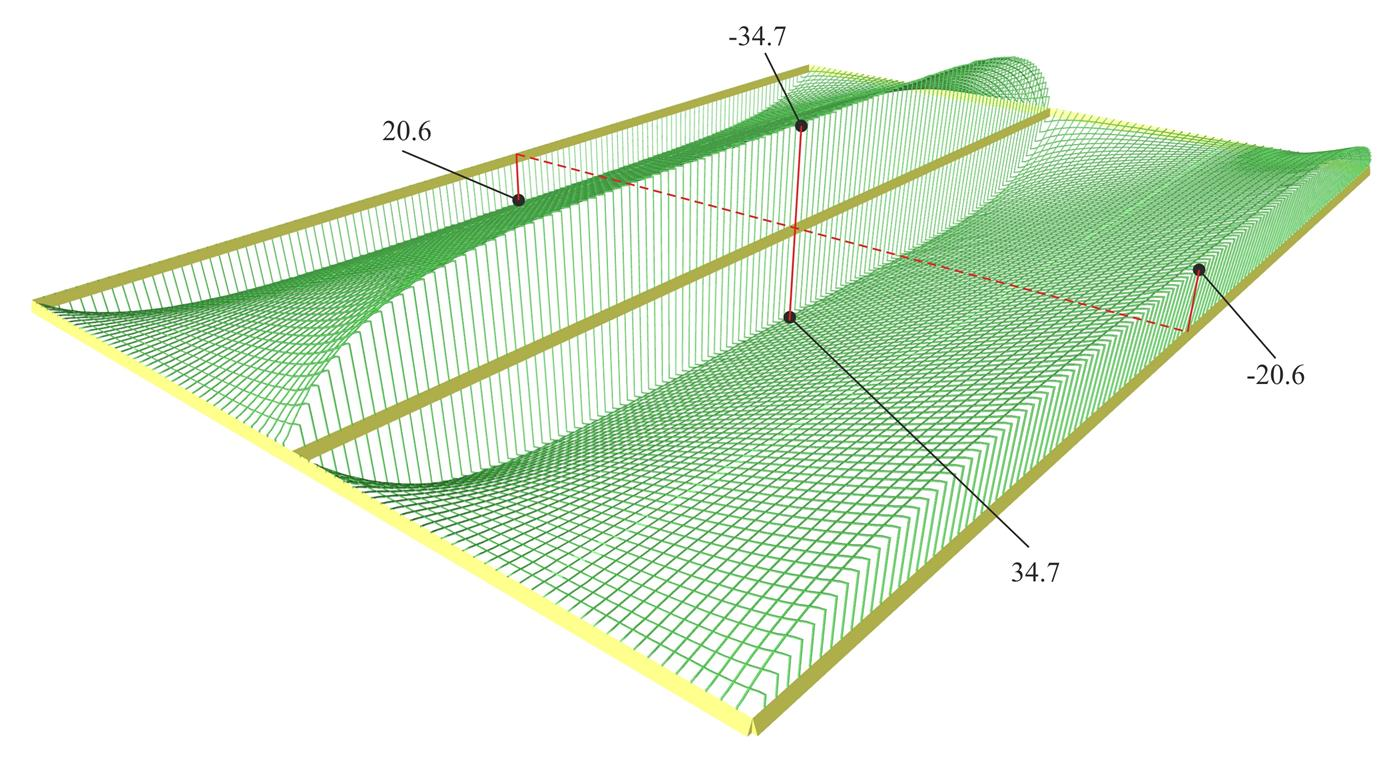Figure 4.2.2-27: Distribution of shear forces [Vx] ([V11] in FEM Results, detailed per 0.10 m in 3D. In the largest part, it matches the corresponding distribution of one-way slabs.
Figure 4.2.2-27: Distribution of shear forces [Vx] ([V11] in FEM Results, detailed per 0.10 m in 3D. In the largest part, it matches the corresponding distribution of one-way slabs.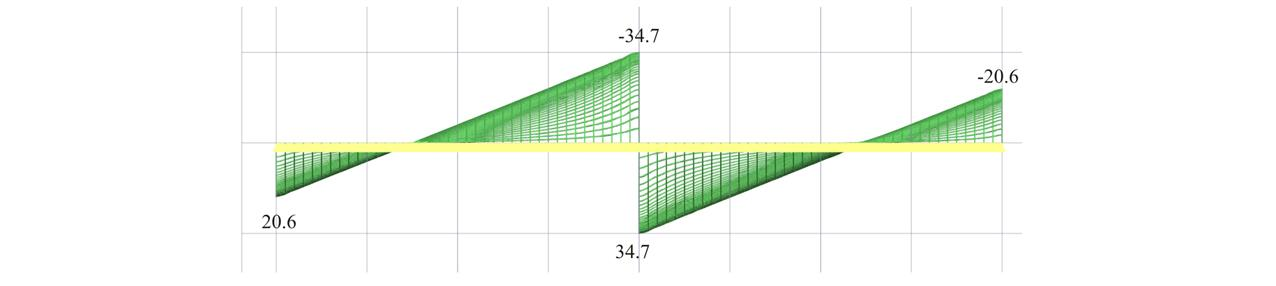Figure 4.2.2-28: Front view of the 3D distribution of shear forces [Vx] ([V11] in FEM Results.
Figure 4.2.2-28: Front view of the 3D distribution of shear forces [Vx] ([V11] in FEM Results.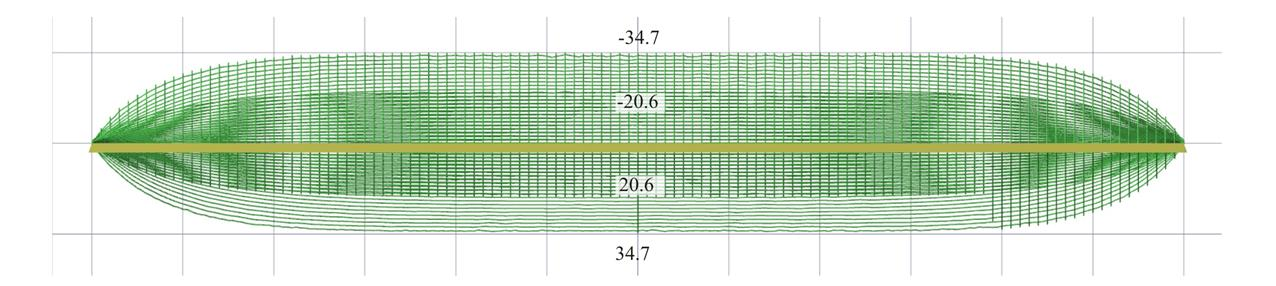Figure 4.2.2-29: Side view of the 3D distribution of shear forces [Vx] ([V11] in FEM Results.
Figure 4.2.2-29: Side view of the 3D distribution of shear forces [Vx] ([V11] in FEM Results.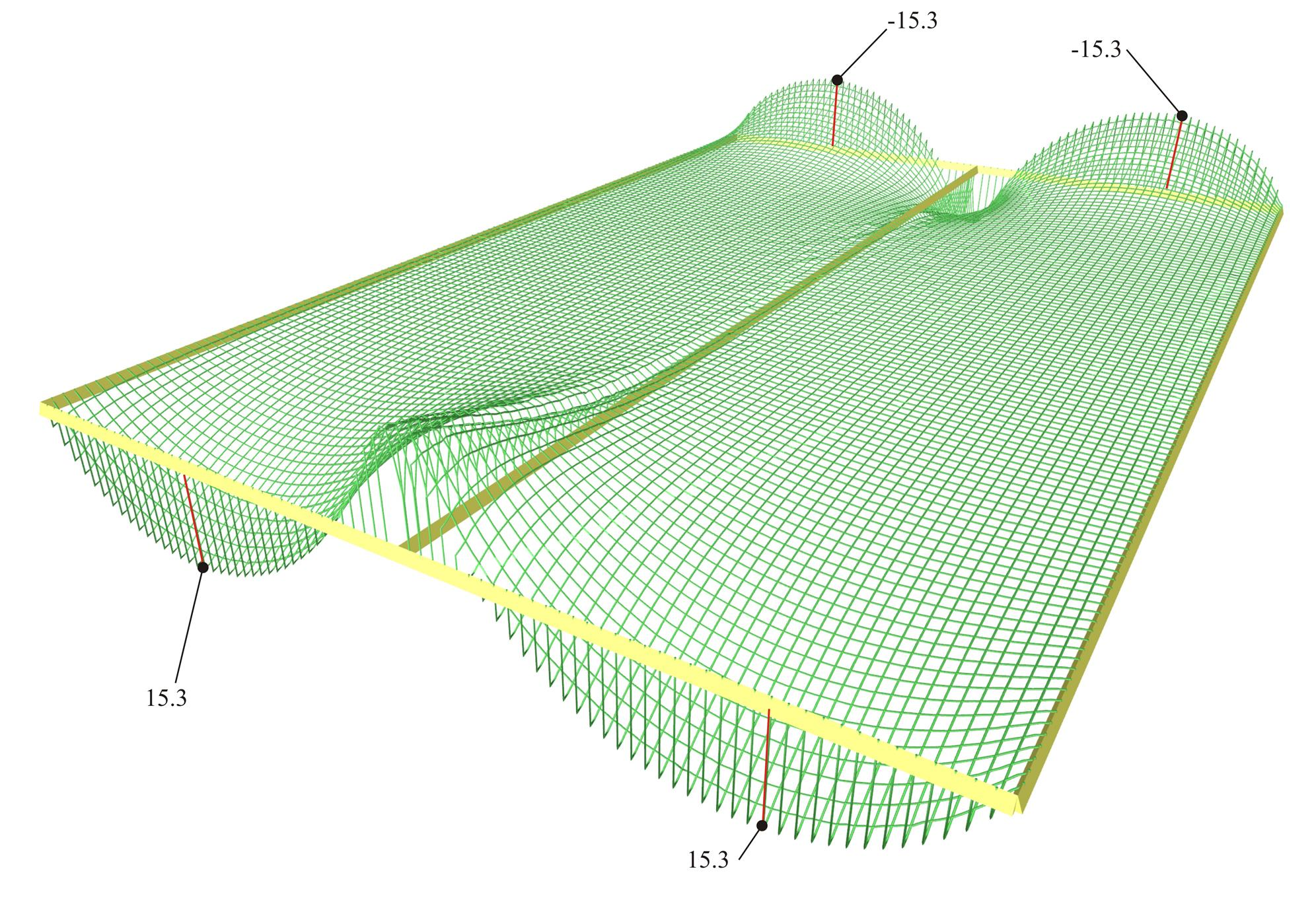Figure 4.2.2-30: Shear forces [Vy] ([V22] in FEM Results, detailed per 0.1 m) extended only to the regions of end supports.
Figure 4.2.2-30: Shear forces [Vy] ([V22] in FEM Results, detailed per 0.1 m) extended only to the regions of end supports.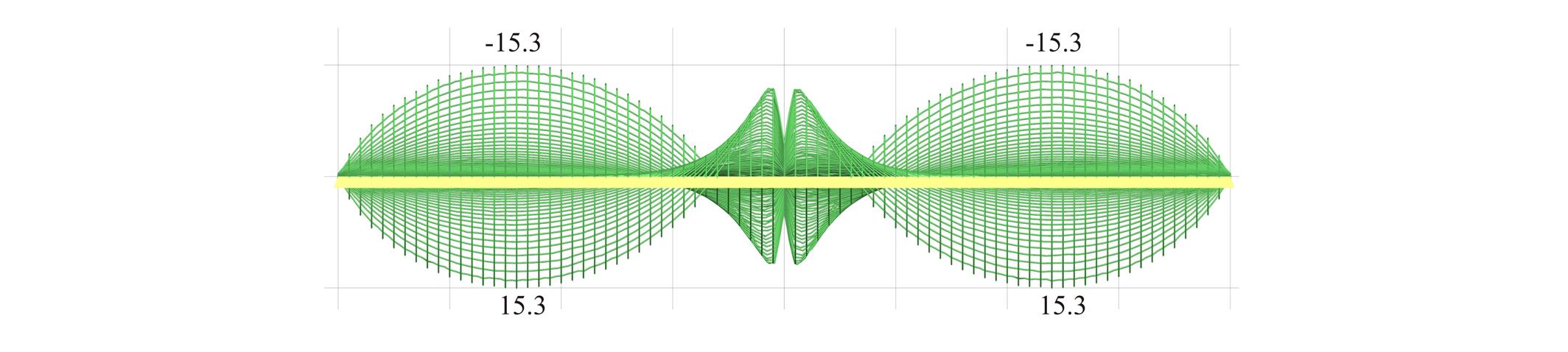Figure 4.2.2-31: Front view of the 3D distribution of shear forces [Vy] ([V22] in FEM Results).
Figure 4.2.2-31: Front view of the 3D distribution of shear forces [Vy] ([V22] in FEM Results).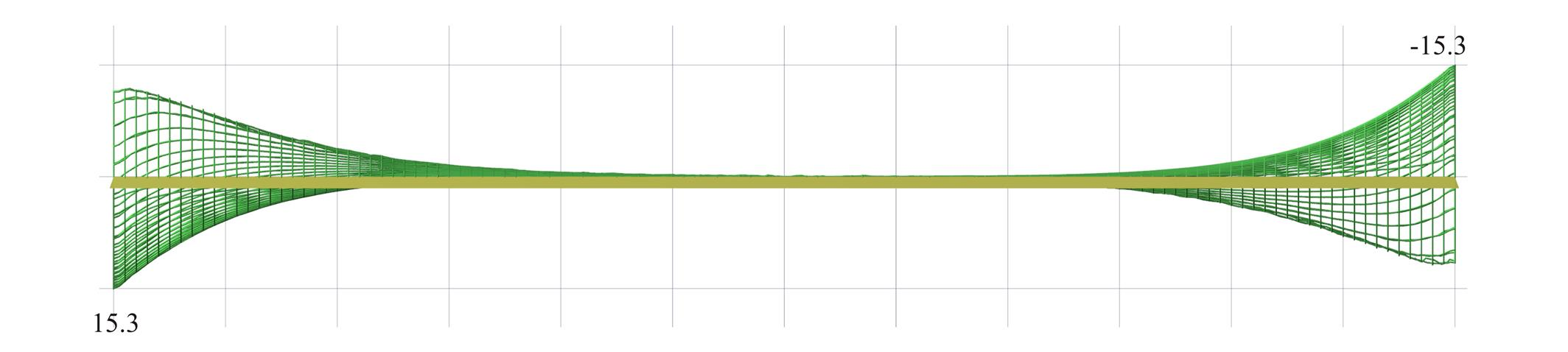Figure 4.2.2-32: Side view of the 3D distribution of shear forces [Vy] ([V22] in FEM Results).
Figure 4.2.2-32: Side view of the 3D distribution of shear forces [Vy] ([V22] in FEM Results).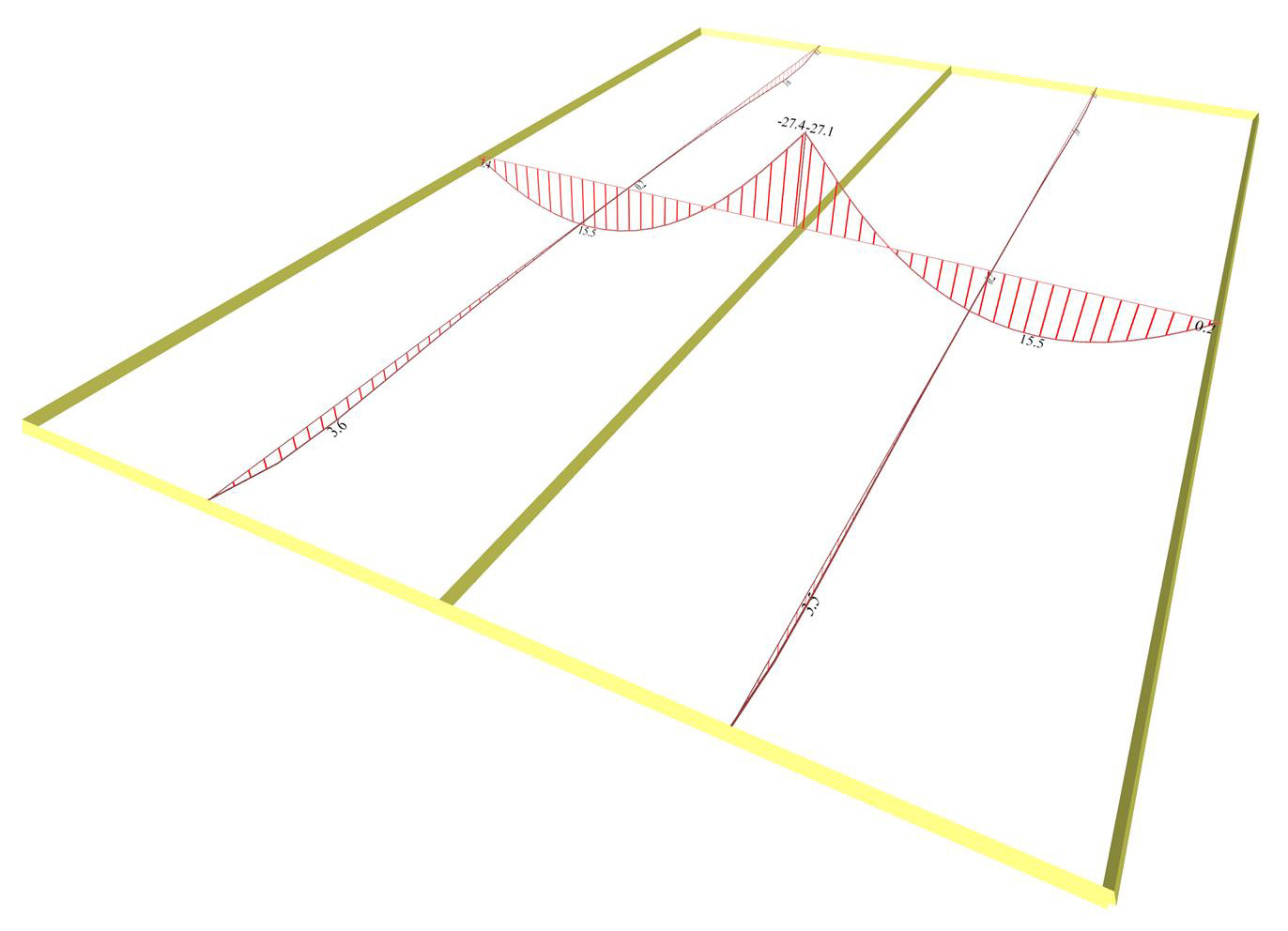Figure 4.2.2-33: The bending moments from Slabs Results
Figure 4.2.2-33: The bending moments from Slabs Results

Notice that in the middle cross-section, the moments [Mx] 15.5 and -27.4 kNm, are equal to the values of the one-way slabs calculated previously.

On the other hand, moments [My] do exist, 3.6 kNm, but they are insignificant.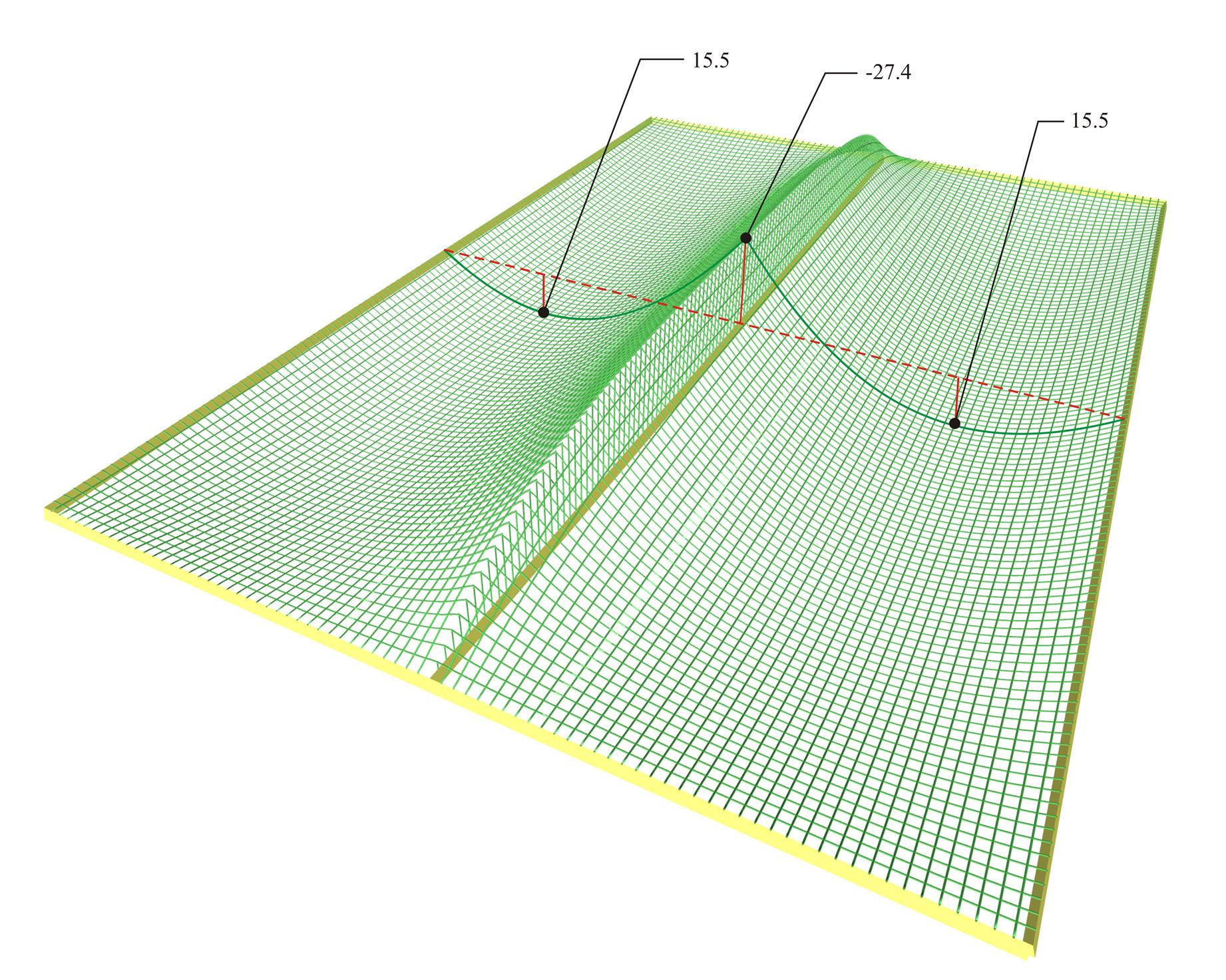Figure 4.2.2-34: Distribution of bending moments [Mx] ([M11] in FEM Results, detailed per 0.10 m) in 3D. Along most of the region, it resembles the corresponding distribution of one-way slabs.
Figure 4.2.2-34: Distribution of bending moments [Mx] ([M11] in FEM Results, detailed per 0.10 m) in 3D. Along most of the region, it resembles the corresponding distribution of one-way slabs.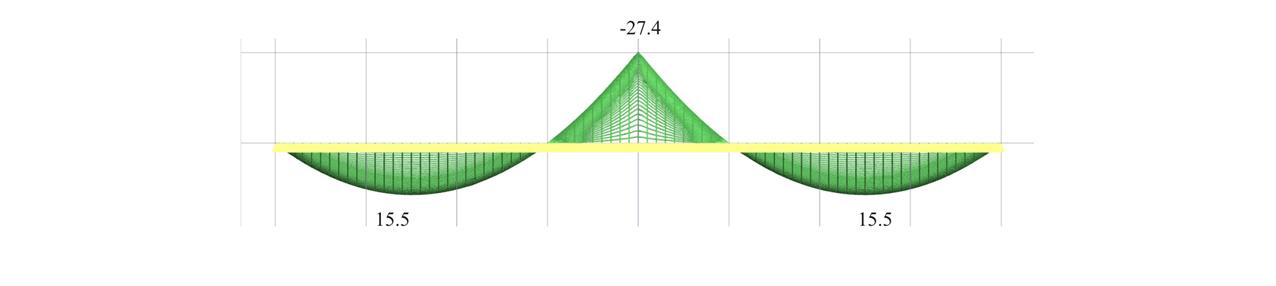Figure 4.2.2-35: Front view of the 3D distribution of bending moments [Mx] ([M11] in FEM Results).
Figure 4.2.2-35: Front view of the 3D distribution of bending moments [Mx] ([M11] in FEM Results).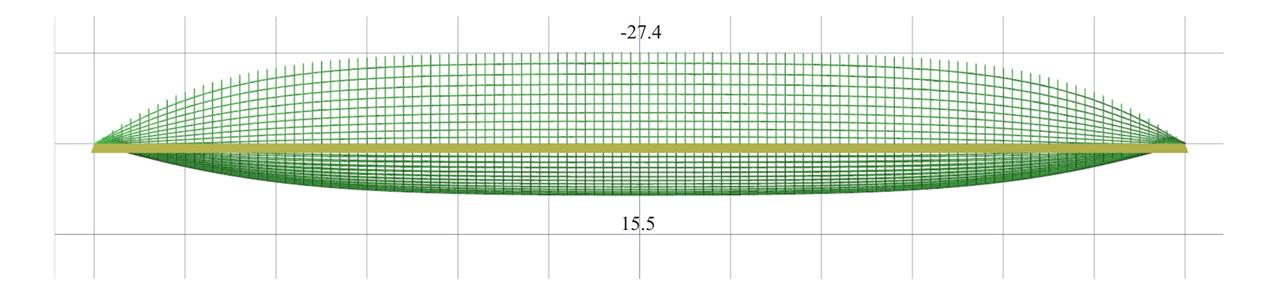Figure 4.2.2-36: Side view of the 3D distribution of bending moments [Mx] ([M11] in FEM Results).
Figure 4.2.2-36: Side view of the 3D distribution of bending moments [Mx] ([M11] in FEM Results).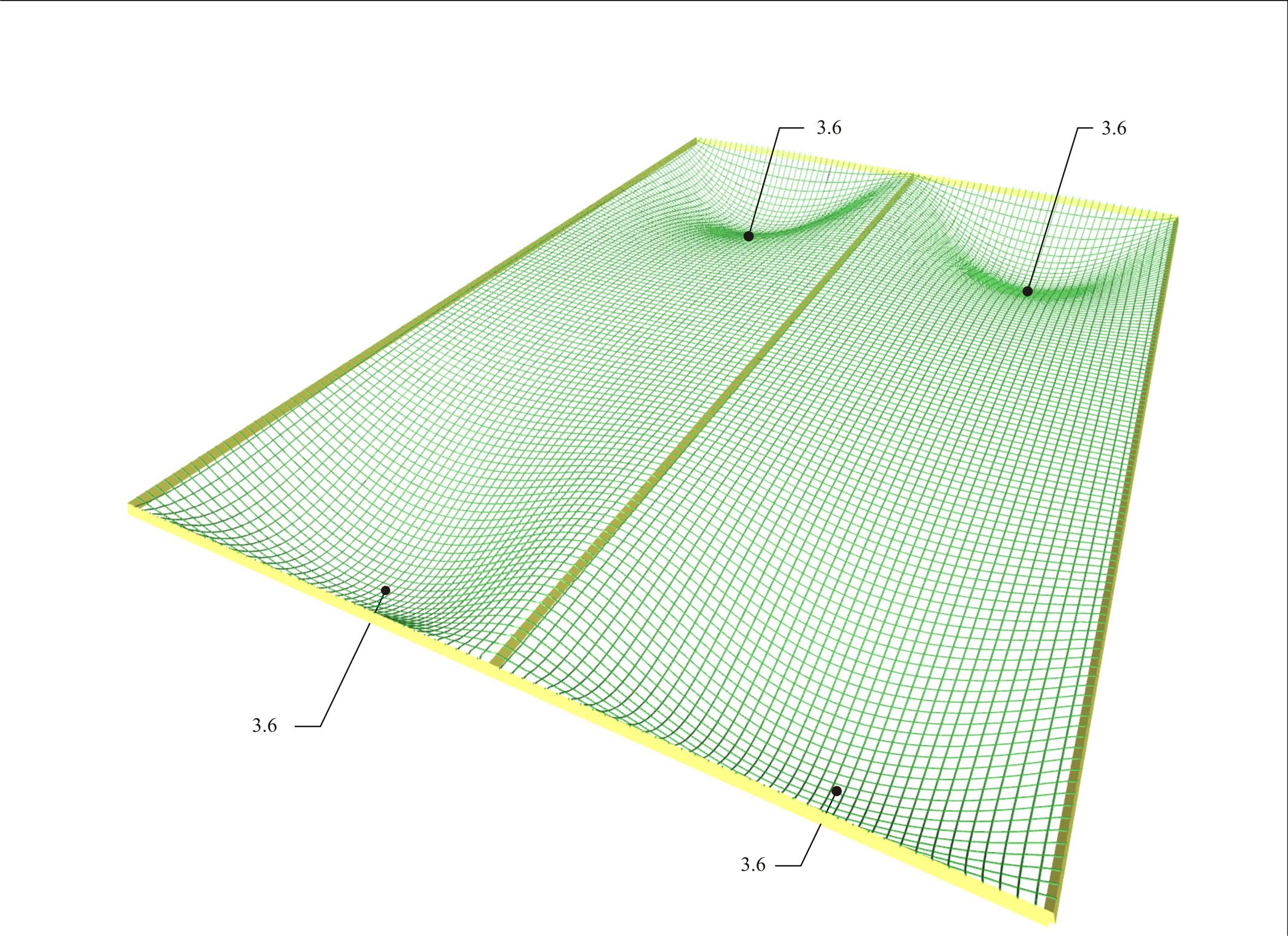Figure 4.2.2-37: Bending moments [My] ([M22] in FEM Results, detailed per 0.1 m) are small and extended only in the regions of end supports.
Figure 4.2.2-37: Bending moments [My] ([M22] in FEM Results, detailed per 0.1 m) are small and extended only in the regions of end supports.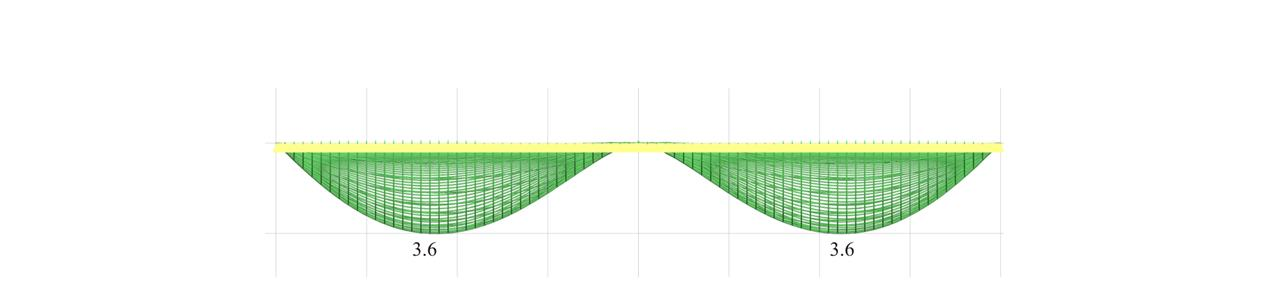Figure 4.2.2-38: Front view of the 3D distribution of the bending moments [My] ([M22] in FEM Results).
Figure 4.2.2-38: Front view of the 3D distribution of the bending moments [My] ([M22] in FEM Results).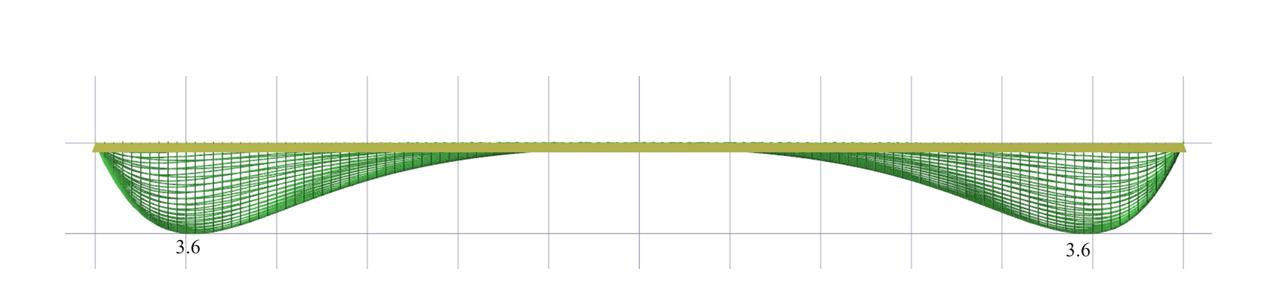Figure 4.2.2-39: Side view of the 3D distribution of the bending moments [My] ([M22] in FEM Results).
Figure 4.2.2-39: Side view of the 3D distribution of the bending moments [My] ([M22] in FEM Results).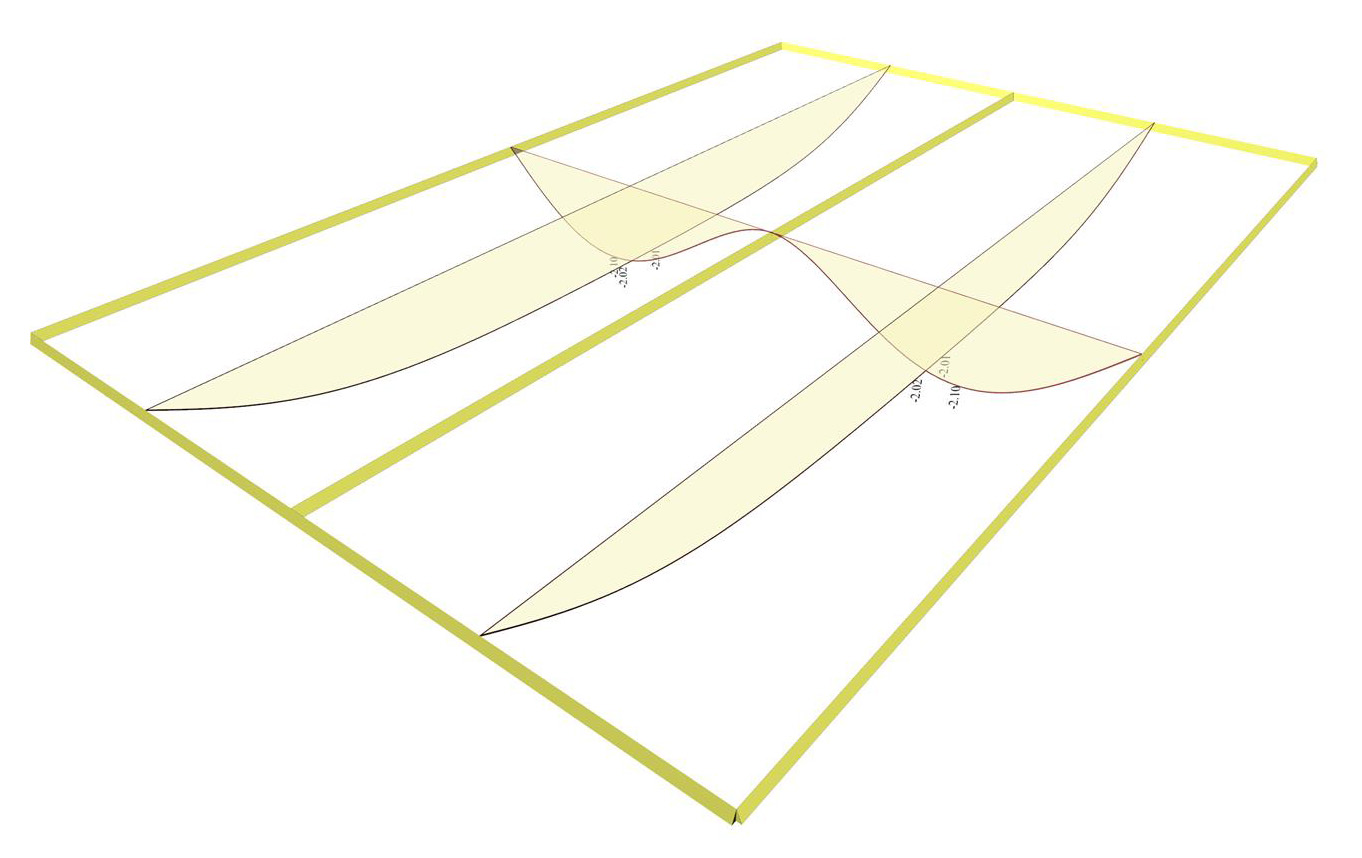Figure 4.2.2-40: Deflection from Slab Results
Figure 4.2.2-40: Deflection from Slab Results

Notice that in the middle cross-section, the deflection is 2.10 mm, that is equal to the respective deflection of the one-way slabs calculated previously.

« Example 4.2.2-1 Example 4.2.2-3 »